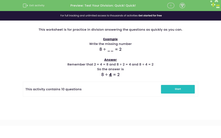# Use Mental Division

In this worksheet, students practise division questions as quickly as possible.Key stage:  KS 2

Curriculum topic:   Number: Multiplication and Division

Curriculum subtopic:   Solve Multiplication/Division Problems

Difficulty level:#### Worksheet Overview

In this activity, we will be learning to divide numbers mentally.

Division and multiplication are the inverses of each other.

This can be really helpful when dividing!

When we know a multiplication fact, we can use this to find two related division facts.Let's try an example together.

Example

Write the missing number

8 ÷ __ = 2

We know that 2 × 4 = 8

So, using the inverse we can say that 8 ÷ 2 = 4 and 8 ÷ 4 = 2

8 ÷ 4 = 2

Now you know how to use related facts, it's time to try some more questions like this.

### What is EdPlace?

We're your National Curriculum aligned online education content provider helping each child succeed in English, maths and science from year 1 to GCSE. With an EdPlace account you’ll be able to track and measure progress, helping each child achieve their best. We build confidence and attainment by personalising each child’s learning at a level that suits them.

Get started